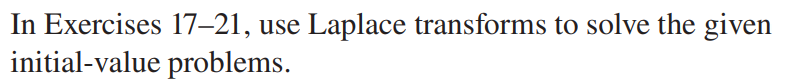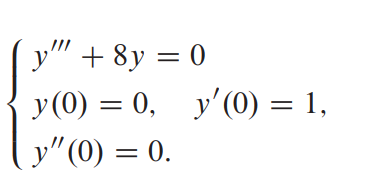# Question In Exercises 17-21, use Laplace transforms to solve the given initial-value problems.>$$\left\{\begin{array}{l}y^{\prime \prime \prime}+8 y=0 \\ y(0)=0, \quad y^{\prime}(0)=1 \\ y^{\prime \prime}(0)=0\end{array}\right.$$TICMRF The Asker · Applied Mathematics
In Exercises 17-21, use Laplace transforms to solve the given initial-value problems.>$$\left\{\begin{array}{l}y^{\prime \prime \prime}+8 y=0 \\ y(0)=0, \quad y^{\prime}(0)=1 \\ y^{\prime \prime}(0)=0\end{array}\right.$$Transcribed Image Text: In Exercises 17-21, use Laplace transforms to solve the given initial-value problems.>$$\left\{\begin{array}{l}y^{\prime \prime \prime}+8 y=0 \\ y(0)=0, \quad y^{\prime}(0)=1 \\ y^{\prime \prime}(0)=0\end{array}\right.$$
More
Transcribed Image Text: In Exercises 17-21, use Laplace transforms to solve the given initial-value problems.>$$\left\{\begin{array}{l}y^{\prime \prime \prime}+8 y=0 \\ y(0)=0, \quad y^{\prime}(0)=1 \\ y^{\prime \prime}(0)=0\end{array}\right.$$